×

Search anything:

# ResNeXt architecture

#### Machine Learning (ML)Get this book -> Problems on Array: For Interviews and Competitive Programming

In this article, we have explored the ResNeXt model architecture which has been developed by Facebook. We have provided a Python implementation of ResNeXt along with applications of the model.

1. Introduction
2. ResNeXt architecture
3. Implementation of ResNeXt
4. Applications of ResNeXt

Pre-requisite:

## Introduction

Image classification refers to the process of categorizing images into classes that are most relevant to the image provided.In order to obtain a better prediction of Image classes it was thought that , deepening of layers might result in accuracy increase , but it was observed that the error rate kept on increasing . To solve this problem the ResNet was introduced but it also had some limitations with regards to lowering the error rate, henceforth ResNeXt was introduced.

ResNext is a simple network for image classification, it uses the same block of layers multiple times that contains a set of transformation functions that helps in classifying the image. As the ResNeXt is made up of same repeated blocks , the architecture is homogenous and the hyperparameters are reduced.

## ResNeXt architecture

The ResNeXt architecture is very similar to that of the ResNet , the only difference is the presence of another dimension called the Cardinality dimension.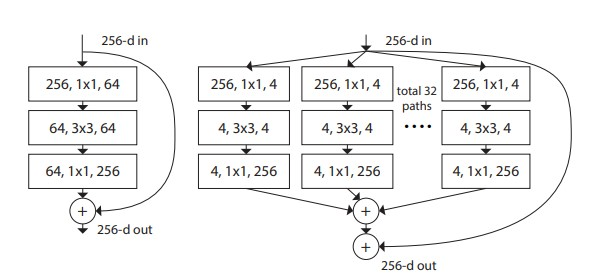As you can see in the figure above , the architecture to the left is of the ResNet and to the right is of the ResNeXt , both the networks use the split-transform-merge strategy. This strategy first splits the input by using the 1X1 convolutional layer into lower dimensions , then transforms it by using the 3x3 or the 5x5 special filter and finally merges it by using the summation operation. The transformations that are being collected come from the same topology , hence making the transformations easy to use without the need of specialized designs.The main objective of the ResNeXt is to handle large inputs and improve the accuracy of the network without the need for building deeper layers but instead increasing the cardinality and hence keeping the complexity relatively simpler.

Simple neuron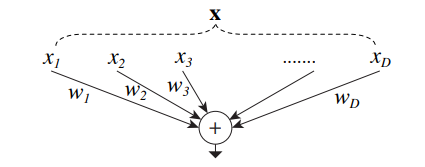The most simple neurons in neural networks work in the way given in the figure above. The inputs [x1,x2,x3....xD] are provided which are further transformed by using the weights [w1,w2,w3....xD] and finally are aggregated using the summation function.

This same concept will be used by the ResNeXt , but instead of the input being concatenated by the weights , the network will be using a more generic function.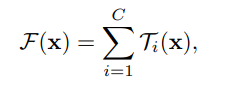The Ti(x) is the generic function we were talking about and the C is the Cardinality of the network, and finally x represents the input.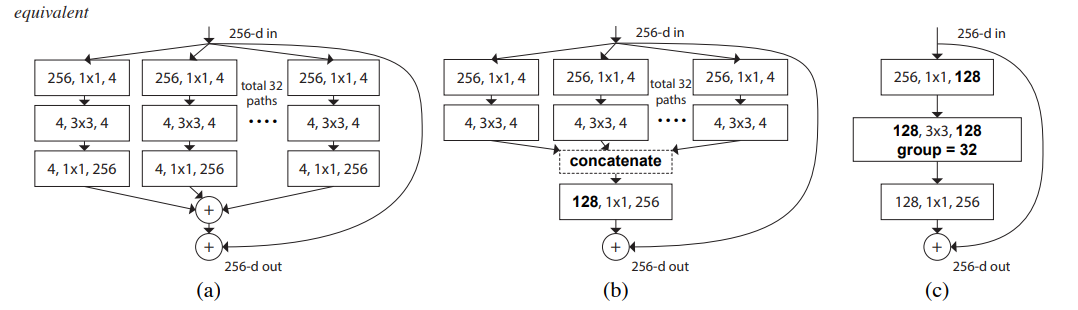The above figure represents the ResNeXt architecture (a) being equivalent to the Inception Resnet (b) and the Grouped Convolution (c).
Compared to (a) , the (b) architecture has early concatenation , meanwhile , the (c) architecture has convolutions grouped.

Relation between cardinality and width: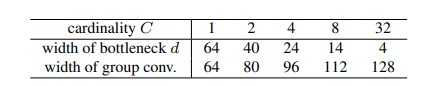As cardinality increases the width of group convolutions also increases , meanwhile width of bottleneck dimension decreases. The relation could be formulated as :
Width of group conv = d * C
Compared to ResNet50 , the ResNext50 displays a lower validation error rate by 1.7 %, due to the Cardinality .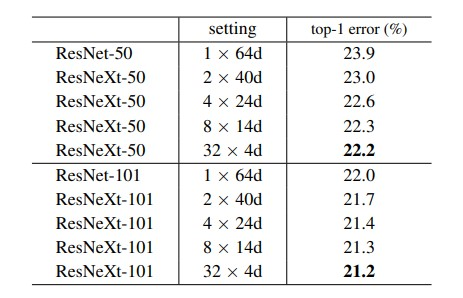In the above figure it is noticeable that , the Increase of layers in ResNeXt decreases the error rate by a small amount and is even more significant when the Cardinality is increased.

## Implementation of ResNeXt

As for the implementation the code is openly available in the github repository, accessible with the link given : facebookresearch/ResNeXt

This is the basic code of implementing the ResNeXt 50 in pytorch and using the torchvision as the tool to access data.

``````import torch
# or
# model = torch.hub.load('pytorch/vision:v0.10.0', 'resnext101_32x8d', pretrained=True)
model.eval()

# sample execution (requires torchvision)
from PIL import Image
from torchvision import transforms
input_image = Image.open(filename)
preprocess = transforms.Compose([
transforms.Resize(256),
transforms.CenterCrop(224),
transforms.ToTensor(),
transforms.Normalize(mean=[0.485, 0.456, 0.406], std=[0.229, 0.224, 0.225]),
])
input_tensor = preprocess(input_image)
input_batch = input_tensor.unsqueeze(0) # create a mini-batch as expected by the model

# move the input and model to GPU for speed if available
if torch.cuda.is_available():
input_batch = input_batch.to('cuda')
model.to('cuda')

output = model(input_batch)
# Tensor of shape 1000, with confidence scores over Imagenet's 1000 classes
print(output)
# The output has unnormalized scores. To get probabilities, you can run a softmax on it.
probabilities = torch.nn.functional.softmax(output, dim=0)
print(probabilities)
``````

The convolutional layer 3,4 and 5 provide as the downsamplers done with striding of 2 . The initial parameters are set as weight decay bein nearly 0.0001 ,momentum as 0.9 and the learning rate is 0.1.

The model arguments are as follows:

• inchannels: input channel dimensionality
• outchannels: output channel dimensionality
• stride: conv stride. Replaces pooling layer.
• cardinality: num of convolution groups.
• basewidth: base number of channels in each group.
• widenfactor: factor to reduce the input dimensionality before convolution.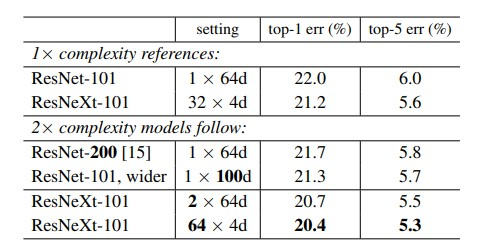The above table shows that the cardinality plays a very major role in increasing the accuracy and hence satisfies the objective of the paper to establish cardinality as an accuracy increaser. More importantly the cardinality dimension eliminates the higher and profound use of depth , which infact increases the error rate.

## Applications of ResNeXt

Applications of ResNeXt are:

• The ResNeXt model is currently being used as a classifier in many microscopic organism classification. The model predicts the class of the organism with an accuracy of 98.45% and F1 score of 0.98.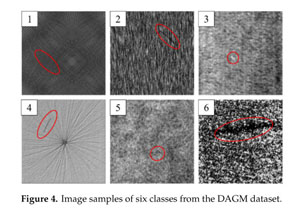• Since the input image accepted by image is of default size 224 X 224 , hence image classification for microscopic objects is more prominent and hence in covid infection detection , it is quite helpful with 3 class and 5 class accuracies being 100% and 98.1% and overall accuracy reaching uptil 75.23 to 81 percentile.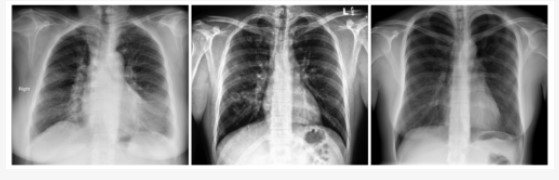With this article at OpenGenus, you must have the complete idea of ResNeXt model architecture.

#### Anubhav Tewari

Anubhav Tewari has been a Machine Learning Developer, Intern at OpenGenus.

ResNeXt architecture